# Texas Go Math Grade 4 Lesson 8.3 Answer Key Area Models and Partial Products

Refer to our Texas Go Math Grade 4 Answer Key Pdf to score good marks in the exams. Test yourself by practicing the problems from Texas Go Math Grade 4 Lesson 8.3 Answer Key Area Models and Partial Products.

## Texas Go Math Grade 4 Lesson 8.3 Answer Key Area Models and Partial Products

Essential Question

How can you use area models and partial products to multiply 2-digit numbers?
Area models help to multiply large numbers, in a simpler forms using multiplication box.
Explanation:
Show the problem as the area of a rectangle, and then break that rectangle into smaller chunks for easier solving.
This method is also known as box multiplication.
Next, multiply to find the area of each smaller rectangle.Investigate

Materials

• color pencilsHow can you use a model to break apart factors and make them easier to multiply?
Break apart factor in multiplication is known as Distributive Property.
Explanation:
The Distributive Property, the Break Apart helps to solve problems by “breaking apart” difficult numbers into easier, more friendly numbers. When we break one of the factors up, but still multiply it, you’ll still get the same product.

A. Outline a rectangle on the grid to model 13 × 18. Break apart the model into smaller rectangles to show factors broken into tens and ones. Label and shade the smaller rectangles. Use the colors below.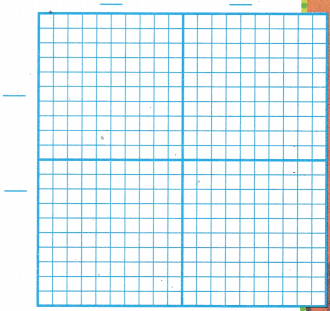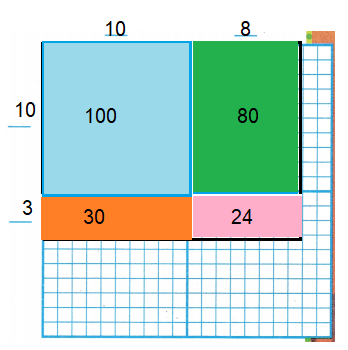100 + 80 + 30 + 24 = 234
Explanation:
Draw outline of a rectangle on the grid to model 13 × 18.
Break apart the model into smaller rectangles to show factors broken into tens and ones.
Then add all together to get the product.

B. Find the product of each smaller rectangle. Then, find the sum of the partial products. Record your answers.100 + 80 + 30 + 24 = 234
Explanation:
First find the product of each smaller rectangle.
Then, find the sum of the partial products.

C. Draw the model again. Break apart the whole model to show factors different from those shown the first time. Label and shade the four smaller rectangles and find their products. Record the sum of the partial products to represent the product of the whole model.
_________ + _________ + _________ + _________ = _________
72 + 72 + 45 + 45 = 234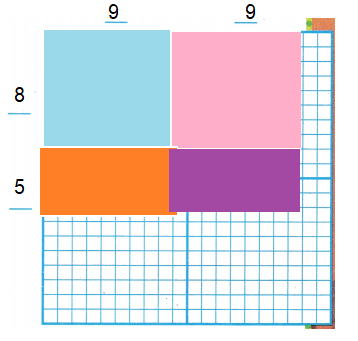Explanation:
Draw outline of a rectangle on the grid to model 13 × 18.
Break apart the model into smaller rectangles to show factors broken into tens and ones.
Then add all together to get the product.
9×8 = 72
9×8=72
5×9 = 45
5×9=45
72+72+45+45= 234

Math Idea
A perfect square is a number that has the same number as its two factors. 144 is a perfect square since both of its factors are 12.
12 x 12 = 144Explanation:
Draw outline of a rectangle on the grid to model 13 × 18.
Break apart the model into smaller rectangles to show factors broken into tens and ones.
Then add all together to get the product.
12 x 12 = 144

Make Connections

You can draw a simple diagram to model and break apart factors to find a product. Find 15 × 15.

STEP 1, Draw a model to show 15 × 15. Break apart the factors into tens and ones to show the partial products.15 x 15 = 225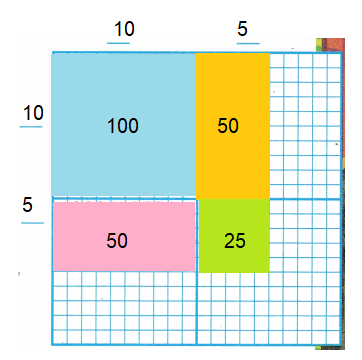Explanation:
Draw outline of a rectangle on the grid to model 13 × 18.
Break apart the model into smaller rectangles to show factors broken into tens and ones.
Then add all together to get the product.
12 x 12 = 144

STEP 2 Write the product for each of the smaller rectangles.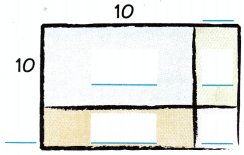STEP 3 Add to find the product for the whole model.
So, 15 × 15 = 225.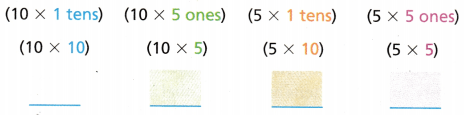Explanation:The model shows four parts.
Each part represents a partial product.
The partial products are 100, 50, 50, and 25.

Math Talk

Mathematical Processes
Explain how breaking apart the factors of a perfect square into tens and ones makes finding the product easier.
One can tell a number is a perfect square by finding its square roots.
Finding the square root is the inverse (opposite) of squaring a number.
If you find the square root of a number and it’s a whole integer, that tells you that the number is a perfect square.
For instance, the square root of 25 is 5.

Share and Show

Find the Product.

Question 1.
16 × 19 = ___________Explanation:
STEP 3 Add to find the product for the whole model.
So, 16 × 19 =304
(10 x 1 tens)  ( 10 x 9 ones )  (6 x 1 tens)  ( 6 x 9 ones )
( 10 x 10 )      ( 10 x 9)           (6 x 10 )          ( 6 x 9 )
100 + 90 + 60 + 54 = 304

Question 2.
18 × 26 = ___________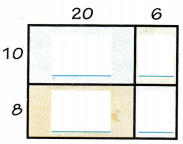Explanation:
Add to find the product for the whole model.
So, 18 × 26 =468
(10 x 2 tens)  ( 10 x 6 ones )  (8 x 2 tens)  ( 8 x 6 ones )
( 10 x 20 )      ( 10 x 6)           (8 x 20 )          ( 8 x 6 )
200 + 60 + 160 + 48 = 468

Question 3.
27 × 39 = ___________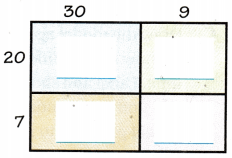Explanation:
Add to find the product for the whole model.
So, 27 × 39 = 1053
(20 x 3 tens)  ( 20 x 9 ones )  (7 x 3 tens)  ( 7 x 9 ones )
( 20 x 30 )      ( 20 x 9)           (7 x 30 )          ( 7 x 9 )
600 + 180 + 210 + 63 = 1053

Question 4.
Evaluate To find the product of 10 and 33, which is the easier computation, (10 × 11) + (10 × 11) + (10 × 11) or (10 × 30) + (10 × 3)? Explain.
Explanation:
first method is
(10 × 11) + (10 × 11) + (10 × 11)
110+110+110 = 330
second method is
(10 × 30) + (10 × 3) = 300 + 30 = 330
second method  is easier
Problem Solving

Multi-Step Sense or Nonsense?

Question 5.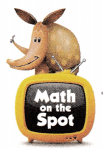Jamal and Kim used different ways to solve 12 × 15 by using partial products. Whose answer makes sense? Whose answer is nonsense? Explain your reasoning.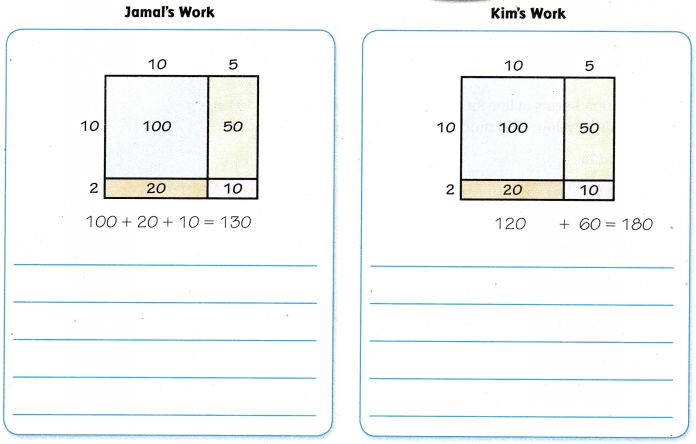Explanation:a. For the answer that is nonsense, write an answer that makes sense.
Explanation:b. H.O.T. Use Diagrams Look at Kim’s method. Can you think of another way Kim could use the model to find the product? Explain.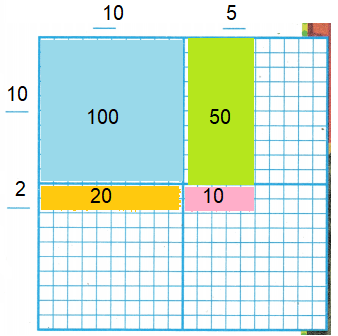Explanation:
Draw outline of a rectangle on the grid to model 12 × 15.
Break apart the model into smaller rectangles to show factors broken into tens and ones.
Then add all together to get the product.
12 x 15 = 180

Question 6.
A restaurant buys 21 cube-shaped watermelons. Each watermelon costs $82. How much does the restaurant pay for the watermelons? (A)$1,720
(B) $1,680 (C)$1,722
(D) $1,640 Answer: Option(C) Explanation: A restaurant buys 21 cube-shaped watermelons. Each watermelon costs$82.
Total amount paid for watermelons by the restaurant
21 x 82 = $1,722 Question 7. There are 14 cars in line for a car wash. A car wash costs$13. Use the model below. How much does it cost to wash all of the cars?(A) $170 (B)$182
(C) $142 (D)$172
Option(B)
Explanation:
There are 14 cars in line for a car wash.
A car wash costs $13. Total cost to wash all of the cars 14 x 13 =$182

Question 8.
Multi-Step Students sell muffins at a school bake sale. They have 12 muffins in each of 15 boxes. They sell 125 muffins. How many muffins are left?
(A) 65 muffins
(B) 5 muffins
(C) 45 muffins
(D) 55 muffins
option(D)
Explanation:
Students have 12 muffins in each of 15 boxes.
Total muffins 12 x 15 = 180
They sell 125 muffins.
Number of muffins left 180 – 125 = 55

TEXAS Test Prep

Question 9.
The cost of a ski-lift ticket is $31. How much will 17 tickets cost? (A)$48
(B) $527 (C)$310
(D) $217 Answer: Option(B) Explanation: The cost of a ski-lift ticket is$31.
Cost of 17 tickets = 31 x 17 = \$527

### Texas Go Math Grade 4 Lesson 8.3 Homework and Practice Answer Key

Find the Product

Question 1.
14 × 18 = _________Explanation:
Add to find the product for the whole model.
So, 14 × 18 = 252
(10 x 1 tens)  ( 10 x 8 ones )  (4 x 1 tens)  ( 4 x 8 ones )
(10 x 10 )      ( 10 x 8)           (4 x 10 )       ( 4 x 8 )
100 + 80 + 40 + 32 = 252

Question 2.
32 × 16 = _________Explanation:
Add to find the product for the whole model.
So, 32 × 16 = 512
(30 x 1 tens)  ( 30 x 6 ones )  (2 x 1 tens)  ( 2 x 6 ones )
(30 x 10 )      ( 30 x 6)            (2 x 10 )       ( 2 x 6 )
300 + 180 + 20 + 12 = 512

Question 3.
26 × 22 = _________Explanation:
Add to find the product for the whole model.
So, 26 × 22 = 572
(20 x 2 tens)  ( 20 x 2 ones )  (6 x 2 tens)  ( 6 x 2 ones )
(20 x 20 )       ( 20 x 2)           (6 x 20 )       ( 6 x 2 )
400 + 40 + 120 + 12 = 572

Problem Solving

Question 4.
Victor and Carla used different ways to solve 37 × 23 by using partial products. Whose answer makes sense? Whose answer is nonsense? Explain your reasoning.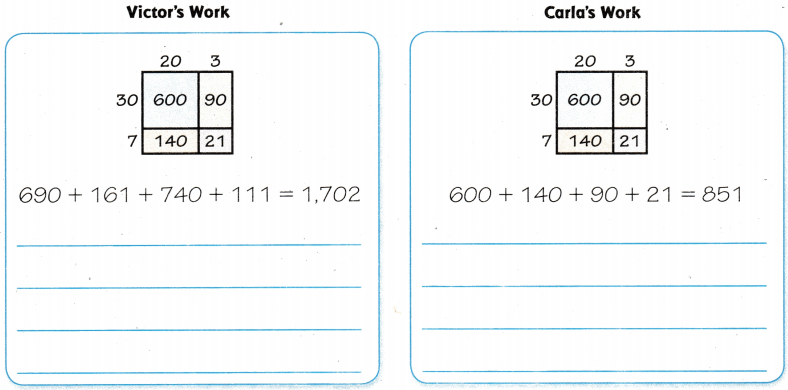Carla’s work is correct
Explanation:
Add to find the product for the whole model.
So, 37 x 23 = 851
(30 x 2 tens)  ( 30 x 3 ones )  (7 x 2 tens)  ( 7 x 3 ones )
(30 x 20 )       ( 30 x 3)           (7 x 20 )       ( 7 x 3 )
600 + 90 + 140 + 21 = 851

Question 5.
For the answer that is nonsense, write an answer that makes sense.
Explanation:
Add to find the product for the whole model.
So, 37 x 23 = 851
(30 x 2 tens)  ( 30 x 3 ones )  (7 x 2 tens)  ( 7 x 3 ones )
(30 x 20 )       ( 30 x 3)           (7 x 20 )       ( 7 x 3 )
600 + 90 + 140 + 21 = 851

Lesson Check

Question 6.
Use the model. What is the product of 17 × 52?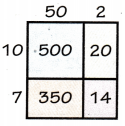(A) 953
(B) 884
(C) 1,768
(D) 1,190
Option(B)
Explanation:
Add to find the product for the whole model.
So, 17 x 52 = 884
(10 x 5 tens)  ( 10 x 2 ones )  (7 x 5 tens)  ( 7 x 2 ones )
(10 x 50 )       ( 10 x 2)           (7 x 50 )       ( 7 x 2 )
500 + 20 + 350 + 14 = 884

Question 7.
Complete the model. What is the product of 44 × 14?(A) 616
(B) 1,120
(C) 1,232
(D) 516
Option(A)
Explanation:
Add to find the product for the whole model.
So, 44 x 11 = 616
(40 x 1 tens)  ( 40 x 4 ones )  (4 x 1 tens)  ( 4 x 4 ones )
(40 x 10 )       ( 40 x 4)           (4 x 10 )       ( 4 x 4 )
400 + 160 + 40 + 16 = 616

Question 8.
A toy store orders 18 boxes of stuffed animals. There are 35 stuffed animals in a box. How many stuffed animals did the store order?
(A) 630
(B) 1,260
(C) 414
(D) 530
Option(A)
Explanation:
A toy store orders 18 boxes of stuffed animals.
There are 35 stuffed animals in a box.
Number of stuffed animals the store order
35 x 18 = 630

Question 9.
Bradley’s heart beats 78 times a minute. How many times does it beat in 45 minutes?
(A) 3,170
(B) 3,210
(C) 3,510
(D) 3,470
Option(C)
Explanation:
Bradley’s heart beats 78 times a minute.
Number of times it beat in 45 minutes
78 x 45 = 3,510

Question 10.
Multi-Step Emma bought 16 packages of rolls for a party. There were 12 rolls in each package. After the party there were 8 rolls left over. How many rolls were eaten?
(A) 192
(B) 138
(C) 200
(D) 184
Option(D)
Explanation:
Emma bought 16 packages of rolls for a party.
There were 12 rolls in each package.
Total rolls in 16 packages
16 x 12 = 192
After the party there were 8 rolls left over.
Number of rolls were eaten 192 -8 = 184

Question 11.
Multi-Step Mr. Ramirez needs 400 paper cups for the school cafeteria. He has 14 packages of paper cups. There are 25 cups in a package. How many more cups does Mr. Ramirez need?
(A) 25
(B) 50
(C) 300
(D) 65